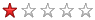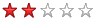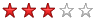Last update: 27/04/2010
General
Simple Nunchaku
Double Nunchaku
Double Nunchaku - Figure eight
There are 7 lesson video(s) in this category.
Videos are sorted by difficulty.

Previous page - 1 - Next page
Double basic rotations
Double basic rotations
Difficulty:Required skills: Basic rebounds and armpit guard, Double upward basic rotation, Double downward basic rotation.
Description: These are basic rotations: this movement should not pose any problem to learn.

Double asynchronous basic rotations
Double asynchronous basic rotations
Difficulty:Required skills: Double upward basic rotation, Double downward basic rotation, Basic rebounds and armpit guard, Double basic rotations.
Description: The principal difficulty here is to desynchronize the movements of the two hands: learn this move slowly at the beginning.

Crossed figure eight
Crossed figure eight
Difficulty:Required skills: Horizontal figure eight normal and advanced.
Description: These are classic figures eight that you have to cross in the middle of the move. To position in offensive guard will enable you to carry out this technique more simply, because the arm which is behind intuitively will pass over the other arm for the crossing part.

Double synchronous normal and reversed figure eight
Double synchronous normal and reversed figure eight
Description: These are classic figures eight that you have to realize with the two hands at the same time.

Figure eight with direction change
Figure eight with direction change
Difficulty:Required skills: Vertical figure eight normal and reversed, Horizontal figure eight normal and advanced, Reversed vertical figure eight with direction change.
Description: This is a sequence of two figures eights with a direction change. No particular difficulty here if you control well this movement in simple Nunchaku.

Double figure eight with direction change
Double figure eight with direction change
Description: These are two figures eight with a direction change realized simultaneously. No difficulty if you already control this type of figure eight.

Description : Movement very similar to the double figure eight with direction change, but this time the eight carried out is not completely the same. The rest of the move is identical.
Close the video

Previous page - 1 - Next page